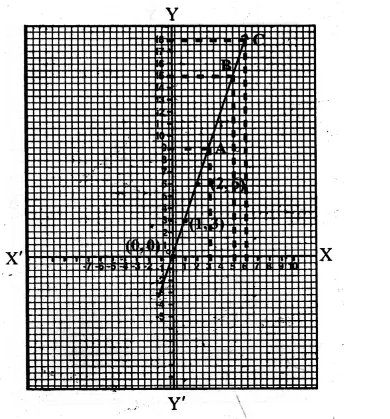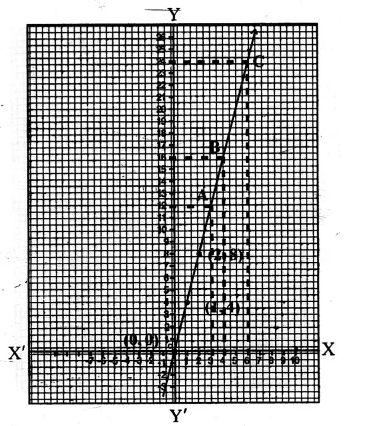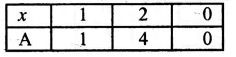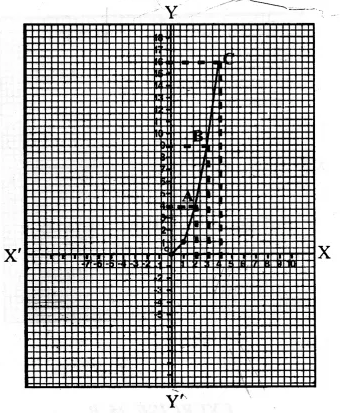## RS Aggarwal Class 8 Solutions Chapter 25 Graphs Ex 25B

These Solutions are part of RS Aggarwal Solutions Class 8. Here we have given RS Aggarwal Solutions Class 8 Chapter 25 Graphs Ex 25B.

Other Exercises

Question 1.
Solution:
(a) y = 3x
By giving some different values to x, we shall get corresponding values of y.
x = 1 then y = 3 x 1 = 3
if x = 2, then y = 3 x 2 = 6
if x = 0, then y = 3 x 0 = 0Now plotting the points given above, and joining them.
(b) We get a line, From the graph.
(i) When x = 3, then y = 9
(ii) When x = 5, then y = 15
(iii) When x = 6, then y = 18 Ans.Question 2.
Solution:
(a) P = 4x
By giving some different values to x, we get the corresponding values of y or P
If x = 1, then P = 4 x 1 = 4
if x = 2, then P = 4 x 2 = 8
if x = 0, then P = 4 x 0 = 0Plot the points (1, 4), (2, 8) and 0, 0) on the graph and join then to get the graph of P = 4x as shown
(b) From the graph we see that
(i) When x = 3, then P = 12
(ii) When x = 4, then P = 16
(iii) When x = 6, then P = 24 Ans.Question 3.
Solution:
A = x²
giving some values to x, we get corresponding values of y or A
If x = 1, then y or A = (1)² = 1
If x = 2, then y or A = (2)² = 4
If x = 0, then y or A = (0)² = 0Now plot the point (1, 1), (2, 4), (0, 0) on the graph, and join them to get the graph of A = x2 as shown(b) From the graph we see that
(i) When x = 2, then A = 4
(ii) When x = 3, then A = 9
(ii) When x = 4 then A = 16 Ans.

Hope given RS Aggarwal Solutions Class 8 Chapter 25 Graphs Ex 25B are helpful to complete your math homework.

If you have any doubts, please comment below. Learn Insta try to provide online math tutoring for you.